﻿ 基于U-Net的变压器磁场云图预测方法 Transformer Magnetic Field Cloud Map Prediction Method Based on U-Net

Journal of Electrical Engineering
Vol. 10  No. 02 ( 2022 ), Article ID: 52332 , 9 pages
10.12677/JEE.2022.102010

1天津工业大学天津市电工电能新技术重点实验室，天津

2河北工业大学省部共建电工装备可靠性与智能化国家重点实验室，天津Transformer Magnetic Field Cloud Map Prediction Method Based on U-Net

Yanyang Wang1, Liang Jin2

1Tianjin Key Laboratory of Advanced Technology of Electrical Engineering and Energy, Tianjin Polytechnic University, Tianjin

2The State Key Laboratory of Reliability and Intelligence of Electrical Equipment Co-Constructed by Hebei University of Technology, Tianjin

Received: Apr. 7th, 2022; accepted: Jun. 6th, 2022; published: Jun. 13th, 2022ABSTRACT

Finite element analysis and calculation have become the main tools for the performance calculation of electromagnetic devices or systems. However, due to the complex modeling and excessive consumption of computing resources when solving electromagnetic fields by traditional finite element methods. This paper adopts a U-shaped deep convolutional neural network. Network magnetic field cloud map prediction model. Taking the transformer as the research object, the electromagnetic coupling finite element model of the transformer is established. By changing the geometric parameters, materials and excitation information of the transformer, the magnetic field cloud image is calculated as the sample data for neural network training. In order to improve the network prediction performance, the U-Net model is optimized by Taguchi method to determine the optimal model settings. Comparing the U-Net model’s predicted magnetic field cloud map with the finite element calculation results, the U-Net model predicts that the mean square error of each pixel in the magnetic field cloud map is in the range of 0.3%~0.9%, which can well learn from the transformer data set. The mapping relationship between the two can generate high-resolution images, thereby reducing the calculation time, which is of great practical significance for deep learning in predicting the direction of the magnetic field cloud map.

Keywords:Traditional Finite Element Method, Electromagnetic Field, U-Shaped Deep Convolutional Neural Network, Transformer1. 引言

2. 变压器模型的建立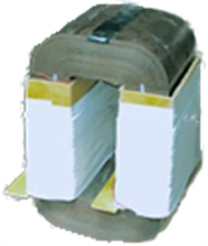Figure 1. Transformer prototype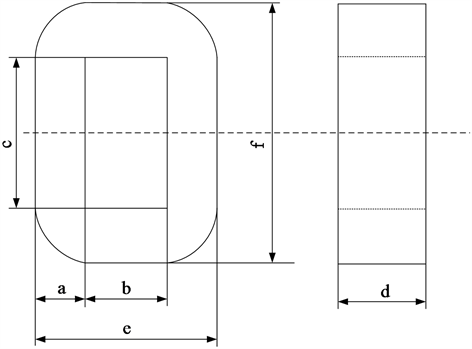Figure 2. The structure of the transformer

3. U型深度卷积神经网络

U型深度卷积神经网络由Ronneberger等人在2015年提出，U-Net网络结构是典型的编码–解码架构的神经网络，如图3所示。U-Net网络模型是FCN模型的改进和延伸，能够使用较小数量的数据完成端到端的训练。具有多层堆叠结构的深度卷积神经网络在图像预测领域已经处于绝对主导地位。

3.1. 编码器

3.2. 解码器

U-Net模型解码器部分，首先采用卷积核大小为2 × 2且滑动步长为2的反卷积层，对特征图进行上采样，使输出的特征图尺寸增大为原来的2倍，通道数量则减小为原来的一半。随后，将反卷积层的上采样结果通过跳跃连接与编码器对应层级的特征图进行级联并有效融合，可以使解码器直接学习编码器部分卷积层提取到的变压器磁场特征，从而防止多次池化下采样使部分磁场特征丢失而影响磁场的预测精度。最后，利用一个3 × 3卷积层对融合后的特征图进一步提取磁场信息，在网络结构末端使用1 × 1的卷积层，仅改变特征图的通道数量，而不改变特征图的尺寸。末端的两个卷积层分别采用了ReLU激活函数和Sigmoid激活函数，主要作用是对前面网络层提取到的输入信息进行整合。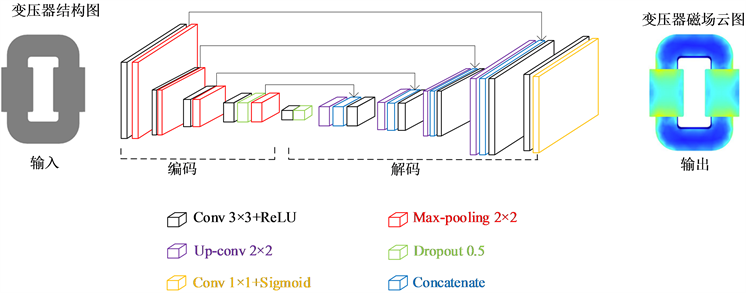Figure 3. U-Net model structure

4. 计算实例

4.1. 数据采集

4.2. 数据预处理

$\stackrel{^}{x}=\frac{x-{x}_{\mathrm{min}}}{{x}_{\mathrm{max}}-{x}_{\mathrm{min}}}$ (1)(a) 变压器结构图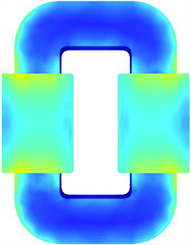(b) 变压器磁场

Figure 4. Sample data set of U-Net model

4.3. 评估指标

$\text{MSE}=\frac{1}{N}\sum _{\text{i=}1}^{m}{\left({\stackrel{^}{y}}_{i}-{y}_{i}\right)}^{2}$ (2)

5. 计算结果及分析

5.1. U-Net模型的超参数调优Table 4. Orthogonal table and model prediction result

5.2. U-Net模型与有限元法性能比较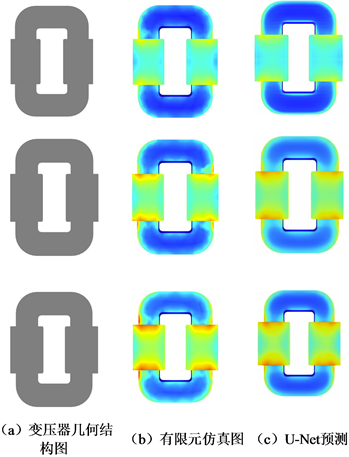Figure 5. Transformer magnetic field cloud map prediction results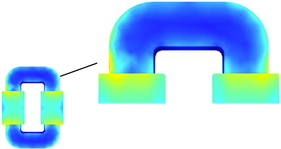(a) 有限元仿真结果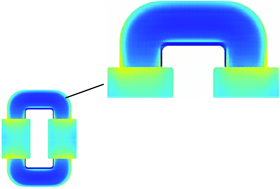(b) U-Net预测结果

Figure 6. Transformer magnetic field cloud map results comparison

6. 结论

Transformer Magnetic Field Cloud Map Prediction Method Based on U-Net[J]. 电气工程, 2022, 10(02): 86-94. https://doi.org/10.12677/JEE.2022.102010

1. 1. 金谋平, 张小林, 王泉. 电磁计算在雷达设计中的应用及需求分析[J]. 电波科学学报, 2020, 35(1): 135-140.

2. 2. Russakovsky, O., Deng, J., Su, H., et al. (2014) ImageNet Large Scale Visual Recognition Challenge. International Journal of Computer Vision, 115, 1-42.

3. 3. Ibtehaz, N. and Rahman, M.-S. (2019) MultiResUNet: Rethinking the U-Net Architecture for Multimodal Biomedical Image Segmentation. Neural Networks, 121, 74-87. https://doi.org/10.1016/j.neunet.2019.08.025

4. 4. 余同瑞, 金冉, 韩晓臻, 等. 自然语言处理预训练模型的研究综述[J]. 计算机工程与应用, 2020, 23(56): 18-28.

5. 5. Liao, L. and Ttig Felix, K. (2016) A Hybrid Framework Combining Data-Driven and Model-Based Methods for System Remaining Useful Life Prediction. Applied Soft Computing, 44, 191-199. https://doi.org/10.1016/j.asoc.2016.03.013

6. 6. Long, J., Shelhamer, E. and Darrell, T. (2015) Fully Convolutional Networks for Semantic Segmentation. 2015 IEEE Conference on Computer Vision and Pattern Recognition (CVPR), Boston, 7-12 June 2015, 3431-3440. https://doi.org/10.1109/CVPR.2015.7298965

7. 7. Khan, A., Ghorbanian, V. and Lowther, D. (2019) Deep Learning for Magnetic Field Estimation. IEEE Transactions on Magnetics, 55, 1-4. https://doi.org/10.1109/TMAG.2019.2899304

8. 8. 林珊, 王红, 齐林海, 等. 基于条件生成对抗网络的短期负荷预测[J]. 电力系统自动化, 2021, 45(11): 52-60.

9. 9. Qi, S., Wang, Y., Li, Y., et al. (2020) Two-Dimensional Electromagnetic Solver Based on Deep Learning Technique. IEEE Journal on Multiscale and Multiphysics Computational Techniques, 5, 83-88. https://doi.org/10.1109/JMMCT.2020.2995811

10. 10. Li, A., Chen, R., Farimani, A.-B., et al. (2020) Reaction Diffu-sion System Prediction Based on Convolutional Neural Network. Scientific Reports, 10, Article No. 3894. https://doi.org/10.1038/s41598-020-60853-2

11. 11. 孟杨. 圆柱绕流及其涡激振动的物理模型与机器学习相结合方法研究[D]: [硕士学位论文]. 大连: 大连海事大学, 2020.

12. 12. Khan, A., Mohammadi, M.-H., Ghorbanian, V., et al. (2020) Efficiency Map Prediction of Motor Drives Using Deep Learning. IEEE Transactions on Magnetics, 56, 1-4. https://doi.org/10.1109/TMAG.2019.2957162

13. 13. 李超然, 肖飞, 樊亚翔, 等. 基于卷积神经网络的锂离子电池SOH估算[J]. 电工技术学报, 2020, 35(19): 4107-4119.

14. 14. 宫文峰, 陈辉, 张美玲, 等. 基于深度学习的电机轴承微小故障智能诊断方法[J]. 仪器仪表学报, 2020, 41(1): 198-208.

15. 15. Ye, S., Zhang, Z., Song, X., et al. (2020) A Flow Feature Detection Method for Modeling Pressure Distribution around a Cylinder in Non-Uniform Flows by Using a Convolutional Neural Network. Scientific Reports, 10, Article No. 4459. https://doi.org/10.1038/s41598-020-61450-z

16. 16. 韩斌, 曾松伟. 基于多特征融合和卷积神经网络的植物叶片识别[J]. 计算机科学, 2021, 48(S1): 113-117.